﻿ Robustness of Quantile Regression to Outliers

Robustness of Quantile Regression to Outliers

Onyedikachi O. JohnOPEN ACCESSPEER-REVIEWED

Robustness of Quantile Regression to Outliers

Onyedikachi O. JohnDepartment of Physical Sciences, Rhema University, Aba

Abstract

Sensitivity of an estimator to departures from its distributional assumptions is a very important issue that is worth considering. The influence function, which describes the effect of an infinitesimal contamination at point, y, on the estimator we are seeking, standardized by the mass, ε, of the contamination, is bounded for the median. This property of the median is enjoyed by the other quantile points. Quantile regression inherits this robustness property since the minimized objective functions in the case of sample quantile and in the case of quantile regression are the same. This robustness is investigated by analyzing the quarterly implicit price deflator using quantile regression. The coefficients for the median and other quantiles remain unchanged even when outlier is added to the data.

• John, Onyedikachi O.. "Robustness of Quantile Regression to Outliers." American Journal of Applied Mathematics and Statistics 3.2 (2015): 86-88.
• John, O. O. (2015). Robustness of Quantile Regression to Outliers. American Journal of Applied Mathematics and Statistics, 3(2), 86-88.
• John, Onyedikachi O.. "Robustness of Quantile Regression to Outliers." American Journal of Applied Mathematics and Statistics 3, no. 2 (2015): 86-88.

 Import into BibTeX Import into EndNote Import into RefMan Import into RefWorks

1. Introduction

An outlier is an extreme observation. Typically, points further than, say, three or four standard deviations from the mean are considered as ‘outliers’. Outliers occur frequently in real data, and can cause one to misinterpret patterns in plots, and may also indicate that model fails to capture the important characteristics of the data. Deleting outliers from the regression model can sometimes give completely different results. Thus the sensitivity of an estimator to departures from its distributional assumptions is a very important issue. The sample mean being a superior estimate of the expectation under normality of the error distribution can be adversely affected even by a single observation if it is sufficiently far from the rest of the data points, Cizek, . On the other hand, the performance of the median can be superior in the presence of outlying observations; a point stressed by many authors including, remarkably, Kolmogorov, .

The modern view of this, strongly influenced by Tukey, , is framed by the sensitivity curve, or influence function of the estimators, and perhaps to a lesser degree by their finite sample breakdown points, . The influence function, introduced by Hampel, , is a population analogue of Tukey’s empirical sensitivity curve. The idea of contaminating a distribution with a small amount of additional data has a long history in statistics and the investigation of robust estimators.

The median has a bounded influence function, implying that the effect of an outlier on a sample median is bounded no matter how far the outlying observation is. This robustness of the median is of course overweighed by lower efficiency in some cases. Other quantiles enjoy similar properties. Quantile regression inherits this robustness property since the minimized objective functions in the case of sample quantiles and in the case of quantile regression are the same.

2. Influence Function

The influence function is the directional derivative of T(F) at F, and it measures the effect of a small perturbation in F on T(F), Essama-Nssah, . Suppose T is a functional of F. ∆y is the probability measure which assigns mass 1 to {y}. The influence function is then defined by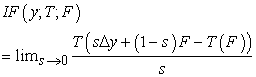(1)

It describes the effect of an infinitesimal contamination at the point, y, on the estimator: in mixed distribution, ε∆y + (1-ε)F, it is as if an observation is randomly sampled from distribution F with probability (1-ε) and from ∆y with probability ε.

A. Influence Function for the Mean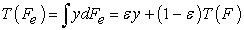(2)

Where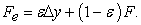So the influence function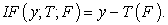(3)

This implies that, as y gets larger, its influence on the mean becomes larger.

B. Influence Function for Quantile Points

For the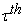quantile points, the influence function,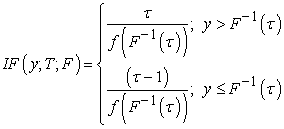(4)

This implies that the influence of contamination at y on the median, and generally on the other quantile points is bounded provided that the sparsity at τ is finite.

C. Influence Function for Quantile Regression

Following the idea expressed in Koenker and Portnoy, , the influence function can be extended to regression. Let F represent the joint distribution of the pairs (x,y). Writing dF in the conditional form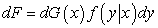(5)

and again assuming that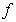is continuous and strictly positive when needed we have,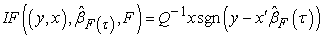(6)

where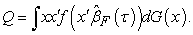Again we see that the estimator has bounded influence in y since y appears only clothed in the protective sgn (·) function. This can also be illustrated in the following theorem, see .

Theorem 1: Let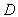be an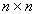diagonal matrix with nonnegative elements and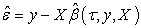be the residual vector of thequantile regression fit with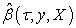thequantile regression estimate of the model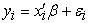,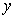the vector of observed dependent variable and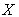the design matrix. Then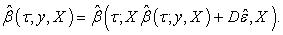(7)

The above theorem indicates that the quantile regression estimate is not affected by any change in the values of the dependent variable for some observations as long as the relative positions of the observation points to the fitted plane are maintained. Intuitively, the breakdown point of an estimator is the proportion of incorrect observations (arbitrarily large observations) an estimator can handle before giving an incorrect (arbitrarily large) result. The higher the breakdown point of an estimator, the more robust it is. The median has a breakdown point of 50%.

3. Analysis and Discussion

To demonstrate the robustness of quantile regression to outlying observations, we consider data from Central Bank of Nigeria, , with the Quarterly Implicit Price Deflator as the dependent variable, and Agriculture, Industry, Building and Construction, Wholesale and Retail, Services, as independent variables. The data cover from 2000 to 2012. R package is used for this analysis, and the result is as follows:

Table 1 and Table 2 give the OLS and quantile regression results for the original data. X1: agriculture, x2: industry, x3: building and construction, x4: wholesale and retail, x5: services.

Table 1. Ordinary Least Squares Estimates

An outlier is added to the data on the implicit price deflator by multiplying an observation by 5, and the resulting data analyzed. Table 3 and Table 4 give the OLS and quantile regression result for this contaminated data.

Again an outlier is added to the original data on the implicit price deflator by multiplying another observation by 7, and the resulting data analyzed. Table 5 and Table 6 respectively give the OLS and quantile regression of the data.

It is clearly seen from the results that the estimates for the conditional mean changed drastically when an outlier is introduced to the original data, giving entirely different representation of the original data, with industry, wholesale and retail, and services showing negative relationship to the implicit price deflator. However, the quantile regression results remain the same even in the presence of the outlying observations.

4. Conclusion

The influence function is an indispensible tool, designed to measure the sensitivity of estimators to infinitesimal perturbation of the nominal model. Quantile regression, introduced by Koenker and Basset, , inherits its robustness property from median regression, and can produce good and reliable estimates even in the presence of extreme outliers.

References

  D. F. Andrew, P. J. Bickel, F. R. Hampel, P. J. Huber, W. H. Roger, J. W. Tukey, “Robust Estimate of Location: survey and advances,” Princeton, Princeton U. Press, 1972.In article  P. Cizek, “Quantile Regression in XploRe Application Guide,” ed. W. Hardle, Z. Hlavka, S. Kline, Berlin, MD Tech Springer, 2003.In article  Central Bank of Nigeria, “2012 Statistical Bulletin: Domestic Product, Consumption and Prices,” Stabull 004, 2013.In article  B. Essama-Nssah and P. J. Lambert, “Influence Functions for Distributional Statistics,” Society for the study of Econoic Inequality, ECINEQ Working Paper Series, 2011.In article  F. Hampel, “The Influence of Curve and its Role in Robust Estimator,” J. of the American Statistical Association, 1974, 69, p.383-393.In article CrossRef  R. Koenker, “Quantile Regression” New York, Cambgridge University Press, 2005,p.138-141In article CrossRef  R. Koenker and G. Basset, “Regression Quantiles” Econometrica, 1978, 46, p.33-50.In article CrossRef  R. Koenker and S. Portnoy, “Quantile Regression,” University of Illinois, Urban Champaign, 1999. Available at http:/www.econ.uiuc.edu/roger/.In article  A. N. Kolmogorov, “The method of the median in the Theory of Errors,” Mat. Sb. Reprinted in selected works of A. N. Kolmogorov, vol II, A. N. Shirayev, (ed), Kluwer: Dordrecht, 1931.In article